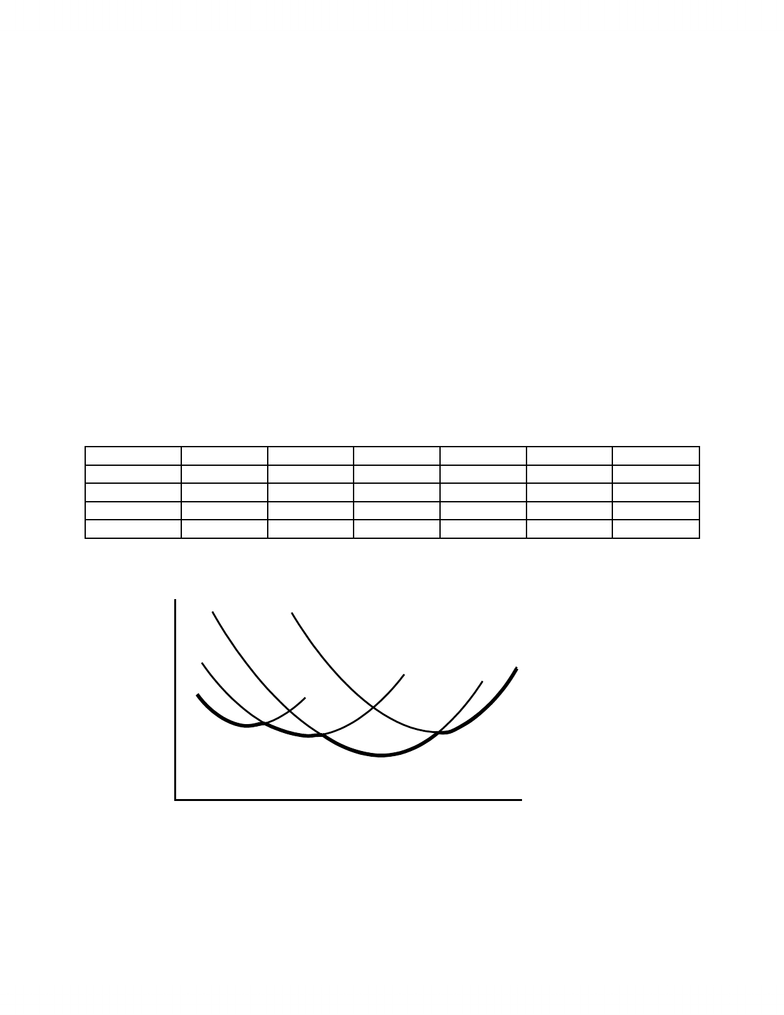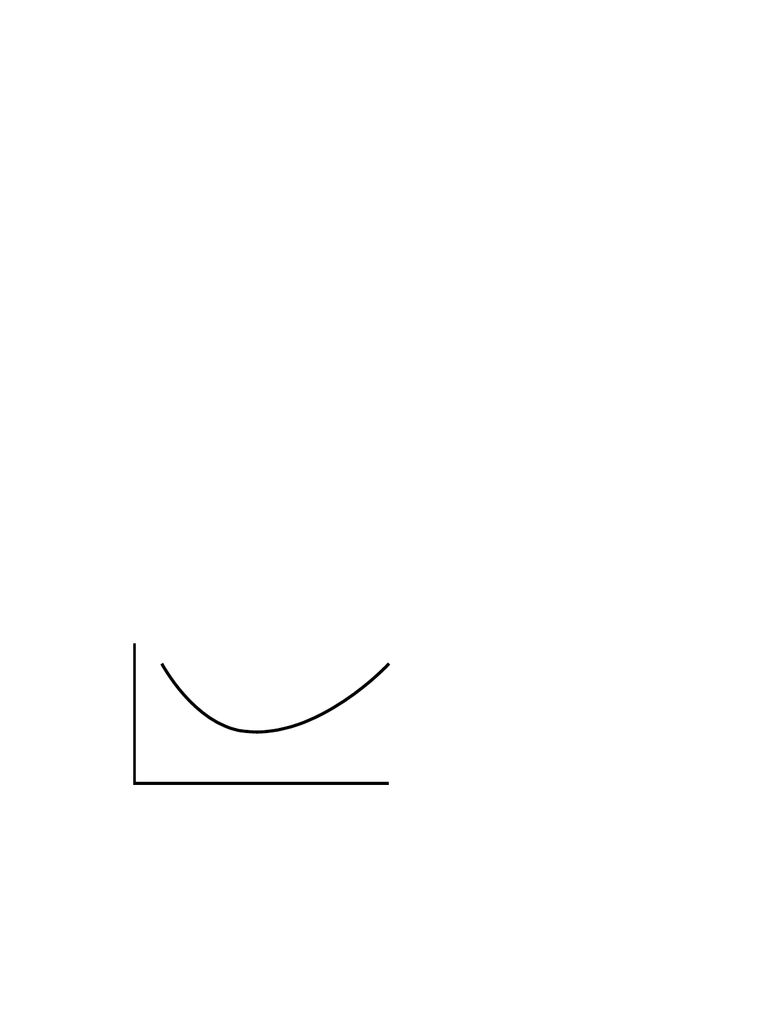# COMMERCE 4FP3 Lecture Notes - Variable Cost, European Cooperation In Science And Technology, Cost Curve

13 views10 pages
School
DepartmentPrinciples of Economics: Long-run Competitive Equilibrium
LONG-RUN COST
Recall that all factors, particularly capital, are variable in the long-run. There are two ways
(plus combinations of the two ways) that Capital can change in the long-run.
1. Change in the size of individual firms through additional or improved equipment and
buildings or through reduction or depreciation of equipment and buildings.
2. Entry into or exit from the industry by firms of the same size as existing firms.
Economies of Scale:
We analyze the effect of changes in the size of individual firm capital through the concept
of economies of scale.
Definition. Economies of Scale (Increasing Returns to Scale) occur when a % increase in all
factor inputs causes a greater % increase in output.
E.g. Suppose that 5 units of Labour and 3 units of Capital produce 100 units of output but that 10
units of Labour and 6 units of Capital produce 250 units of output.
=> 100% increase in factor inputs produces a 150% increase in output.
Why does the concept of Economies of Scale not contradict Eventually Diminishing
Returns (MP)?
Definition: Diseconomies of Scale (Decreasing Returns to Scale) occurs when a % increase in
all factors causes a smaller % increase in output.
Definition: Constant Returns to Scale occurs when a % increase in all factors causes the same
% increase in output.
Average Cost decreases with Economies of Scale
The key element of economies of scale is a decrease in Average Cost with increases in output.
- 1 -
Unlock document

This preview shows pages 1-3 of the document.
Unlock all 10 pages and 3 million more documents.Principles of Economics: Long-run Competitive Equilibrium
Proof: Recall that Average Cost = ACo = (wL + rK)/q
Define ‘a’ as the % change in inputs and ‘b’ as the % change in outputs.
=> AC1 = (waL + raK)/bq = a/b(wL + rK)/q = a/b * ACo
a < b => Economies of Scale (Increasing Returns to Scale) => Average Cost decreases
a = b => Constant Returns to Scale => no change in Average
Cost
a > b => Diseconomies of Scale (Decreasing Returns to Scale) => Average Cost increases
Derivation of Long-run Average Cost from Short-run Average Cost Curves
Since each quantity of capital gives specific short-run cost functions, we can derive long-
run average cost from the short-run average cost function of each capital.
Quantity 20 40 60 80 100 120
ACo (Ko) \$20 \$14 \$10 \$15 \$22 \$30
AC1(K1>Ko) \$30 \$20 \$12 \$8 \$10 \$14
AC2(K2>K1) \$40 \$25 \$15 \$10 \$6 \$8
AC3(K3>K2) \$60 \$45 \$32 \$20 \$14 \$10
Long-run Average Cost
Cost/unit
Quantity
ACo
AC
1
AC
2
AC
3
LRAC
Average Cost falls to minimum and then rises for each Capital. Given that fixed cost is
larger for larger capitals, average cost for small output is greater for larger capitals relative to
- 2 -
Unlock document

This preview shows pages 1-3 of the document.
Unlock all 10 pages and 3 million more documents.Principles of Economics: Long-run Competitive Equilibrium
smaller capitals. Average Cost is eventually lower for larger capitals relative to smaller capitals
if there is economies of scale but minimum average cost will rise if there are diseconomies of
scale.
Note that falling long-run average cost implies economies of scale and rising long-run
average cost implies diseconomies of scale.
Long-run Average Cost tends to initially decrease due to increasing returns to scale with
increased capital and labour and eventually increase due to decreasing returns to scale with
increased capital and labour. There is only one minimum average cost attainable though it may
occur with different amounts of capital and labour. The smallest capital that gives this minimum
average cost is called Minimum Efficient Scale.
Long-run Average Cost (LRAC)
Long-run Average Cost shows the lowest average cost for each output in the long-run. This
defines the size of capital for lowest average cost for each output.
If capital is infinitely divisible, Long-run Average Cost is a smooth curve with each point
from the short-run average cost of each capital.
Average Cost
q
Increasing
Returns
Minimum
Efficient Scale
Decreasing
Returns
Profit Maximization in the long-run implies that firms change capital and labour until they
find the capital with the Short-run Average Cost function that gives minimum Long-run Average
Cost for the desired output.
- 3 -
Unlock document

This preview shows pages 1-3 of the document.
Unlock all 10 pages and 3 million more documents.